# PSAT Math : Rhombuses

## Example Questions

### Example Question #1 : How To Find The Perimeter Of A Rhombus

If the area of a rhombus is 24 and one diagonal length is 6, find the perimeter of the rhombus.

8

12

20

16

24

20

Explanation:

The area of a rhombus is found by

A = 1/2(d1)(d2)

where d1 and d2 are the lengths of the diagonals.  Substituting for the given values yields

24 = 1/2(d1)(6)

24 = 3(d1)

8 = d1

Now, use the facts that diagonals are perpendicular in a rhombus, diagonals bisect each other in a rhombus, and the Pythagorean Theorem to determine that the two diagonals form 4 right triangles with leg lengths of 3 and 4.  Since 32 + 42 = 52, each side length is 5, so the perimeter is 5(4) = 20.

### Example Question #1 : Quadrilaterals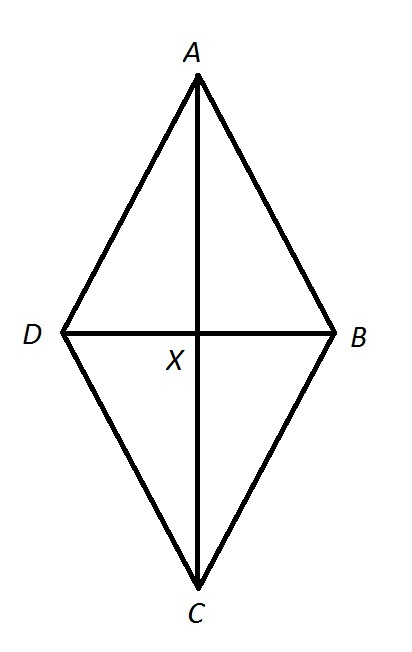Note: Figure NOT drawn to scale.

Calculate the perimeter of Quadrilateralin the above diagram if: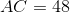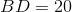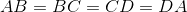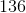Insufficient information is given to answer the question.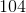Explanation:, so Quadrilateralis a rhombus. Its diagonals are therefore perpendicular to each other, and the four triangles they form are right triangles. Therefore, the Pythagorean theorem can be used to determine the common sidelength of Quadrilateral.

We focus on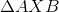. The diagonals are also each other's bisector, so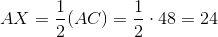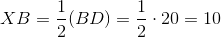By the Pythagorean Theorem,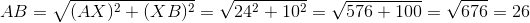26 is the common length of the four sides of Quadrilateral, so its perimeter is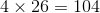.

### Example Question #242 : Geometry

A rhombus has a side length of 5. Which of the following is NOT a possible value for its area?

24

25

10

30

15

30

Explanation:

The area of a rhombus will vary as the angles made by its sides change. The "flatter" the rhombus is (with two very small angles and two very large angles, say 2, 178, 2, and 178 degrees), the smaller the area is. There is, of course, a lower bound of zero for the area, but the area can get arbitrarily small. This implies that the correct answer would be the largest choice. In fact, the largest area of a rhombus occurs when all four angles are equal, i.e. when the rhombus is a square. The area of a square of side length 5 is 25, so any value bigger than 25 is impossible to acheive.

### Example Question #2 : Quadrilaterals

In Rhombus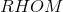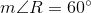. If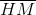is constructed, which of the following is true about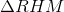?obtuse and scaleneis obtuse and isosceles, but not equilateralis acute and equilateralis acute and isosceles, but not equilateralis acute and scaleneis acute and equilateral

Explanation:

The figure referenced is below.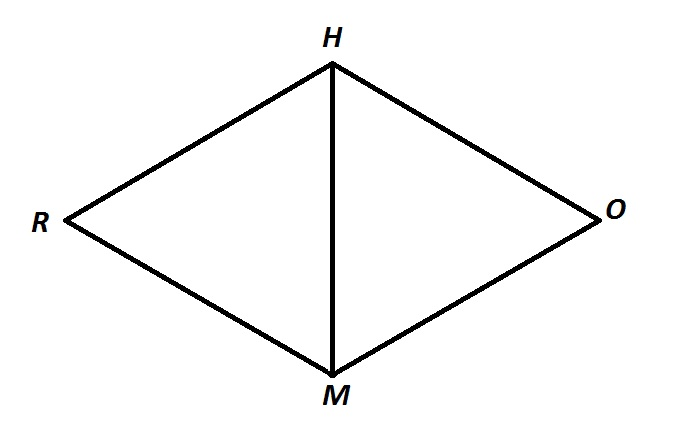Consecutive angles of a rhombus are supplementary - as they are with all parallelograms - so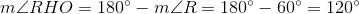A diagonal of a rhombus bisects its angles, so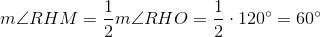A similar argument proves that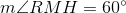.

Since all three angles ofmeasure, the triangle is acute. It is also equiangular, and, subsequently, equilateral.

### Example Question #1 : How To Find An Angle In A Rhombus

In Rhombus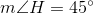. If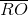is constructed, which of the following is true about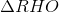?is right and scaleneis acute and scaleneis right and isosceles, but not equilateralis acute and isosceles, but not equilateralis acute and equilateralis acute and isosceles, but not equilateral

Explanation:

The figure referenced is below.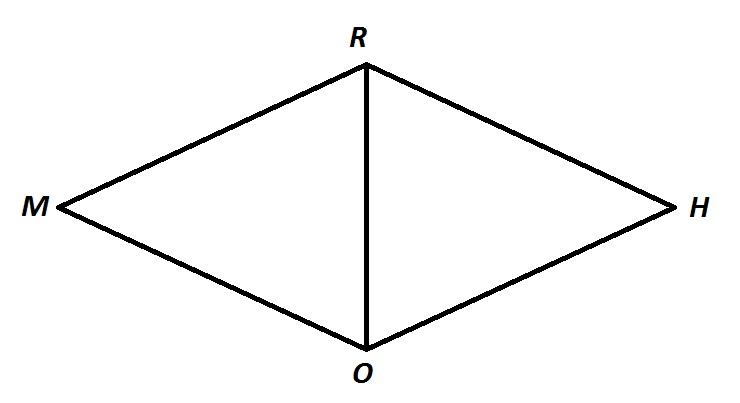The sides of a rhombus are congruent by definition, so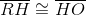, makingisosceles. It is not equilateral, since, and an equilateral triangle must have threeangles.

Also, consecutive angles of a rhombus are supplementary - as they are with all parallelograms - so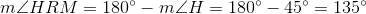A diagonal of a rhombus bisects its angles, so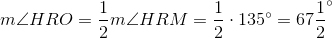Similarly,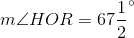This makesacute.

The correct response is thatis acute and isosceles, but not equilateral.